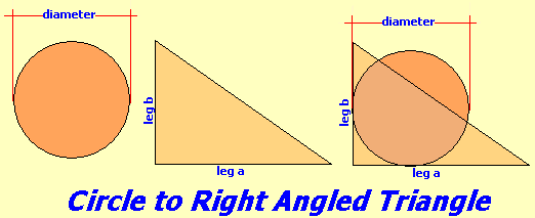Spike's Calculators

# Changing a Circle to a Right Angled Triangle

This calculator converts the area of a circle into a right angled triangle with the same area. The calculation is based on the area of the right angled triangle being the same as the circle's area and the length of one of the legs of this triangle.### Circle Formula's

Area;
`A = π * D² ÷ 4`
where A = area, π = 3.14159..., D = diameter

Circumference;
`C = 2 * π * D ÷ 2`
where C = circumference, π = 3.14159...., D = diameter

### Right Angled Triangle Formula's

Area:
`area = leg a * leg b ÷ 2`

Solving the second leg;
If leg a and the area are known, solving for leg b
`area =  leg a * leg b ÷  2`
rewritten as:
`leg b =  area ÷  leg a * 2`

Hypotenuse
`H = √ leg a² + leg b²`

Perimeter
`P = leg a + leg b + hypotenuse `

### Circle to Right Angled Triangle

diameter of the circle {{selectedunit.m1}}
first leg length {{selectedunit.m1}}
decimal rounding # of digits in results

#### Results:

 circle's radius {{selectedunit.m1}} area of the circle {{selectedunit.m1}}² circumference of the circle {{selectedunit.m1}} second leg length {{selectedunit.m1}} hypotenuse length {{selectedunit.m1}} perimeter of the the right angled triangle {{selectedunit.m1}}

#### Calculation

1. enter the diameter of a circle
2. leg length of the triangle (can be either one)

#### Results

2. the area of the circle
3. the perimeter of the circle (circumference)
4. the second leg's length of the triangle
5. length of the hypotenuse
6. perimeter of the right angled triangle